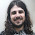## Thursday, April 15, 2010

### how to prove it, ch-1, sec 1.3(Variables and Sets) ex

With variables we can have general statements containing free variables, True/False of which is fixed only when variable is fixed to a value unlike propositional statement whose value is fixed.

Writing a set definition using elementhood test means writing it like {x | P(x)} where P(x) is the desired test/predicate.

In general $y \in \lbrace x \in A | P(x) \rbrace$ means the same thing as $(y \in A) \land P(y)$
=========================================================================================

Ex-1(a) $D(6) \land D(9) \land D(15)$ where D(x) means "x is divisible by 3"

Ex-1(b) $D(x,2) \land D(x,3) \land \lnot D(x,4)$ where D(x,y) means "x is divisible by y"

Ex-1(c) $N(x) \land N(y) \land (P(x) \lor P(y)) \land \lnot (P(x) \land P(y))$ where N(x) means "x is a natutal number" and P(x) means "x is a prime number"

Ex-2(a) $M(x) \land M(y) \land (Taller(x,y) \lor Taller(y,x))$ where M(x) means "x is a Man" and Taller(x,y) means "x is taller than y"

Ex-2(b) $(B(x) \lor B(y)) \land (R(x) \lor R(y))$ where B(x) means "x has brown eyes" and R(x) means "x has red hair"

Ex-2(c)
$(B(x) \land R(x)) \lor (B(y) \land R(y))$ where B(x) means "x has brown eyes" and R(x) means "x has red hair"

Ex-3(a) {x | x is a planet}

Ex-3(b) {x | x is an university}

Ex-3(c) {x | x is a state of USA}

Ex-3(d) {x | x is province or territory in canada}

Ex-4(a) {$x^2$ | $x \in Z+$}

Ex-4(b) {$2^x$ | $x \in N$}

Ex-4(c) {$x \in Z | (x \geq 10) \land (x \leq 19)$}

Ex-5(a) $(-3 \in R) \land (13 - 2*-3 > 1)$ = $(-3 \in R) \land (19 > 1)$
bound variable is 'x', no free variables and statement is True.

Ex-5(b) $(4 \in R-) \land (5 > 1)$
bound variable is 'x', no free variables, but statement is False as $4 \in R-$ is False.

Ex-5(c) $\lnot((5 \in R) \land (3 > c))$
bound variable is 'x', one free variable 'c'

Ex-6(a) $(w \in R) \land (13 - 2w > c)$
bound variable is 'x'; free variables are 'w' and 'c'

Ex-6(b) P(y) means "y is a prime number"
$(4 \in R) \land P(5)$
bound variables are 'x' and 'y'; no free variables; statement is True.

Ex-6(c) P(y) means "y is a prime number"
$P(4) \land (5 > 1)$
bound variables are 'x' and 'y'; no free variables; statement is False

Ex-7(a) {x | x is or was husband of Elizabeth Taylor }

Ex-7(b) $\lbrace \land, \lor, \lnot \rbrace$

Ex-7(c) {"Daniel J. Velleman"}

Ex-8(a) $\lbrace x \in R | x^2 - 4x + 3 = 0 \rbrace$ = {1,3}

Ex-8(b) $\lbrace x \in R | x^2 -2x + 3 = 0 \rbrace$ = $\phi$

Ex-8(c) $\lbrace x \in R | 5 \in \lbrace y \in R | x^2 + y^2 < 50 \rbrace \rbrace$
= {$x \in R | x^2 + 5^2 < 50$}
= {$x \in R | x^2 < 25$}
= {1,2,3,...}

#### 1 comment:

1.First of all, many thanks for solving it entirely (and many years ago). I've been comparing your solutions and strategies (and plan to do so) until I finish the entire book.

I'd also like to point to someone reading the comments that Ex-8(c) might be {x element of R | -5 < x < 5}.

Greetings from Brazil,
Leandro